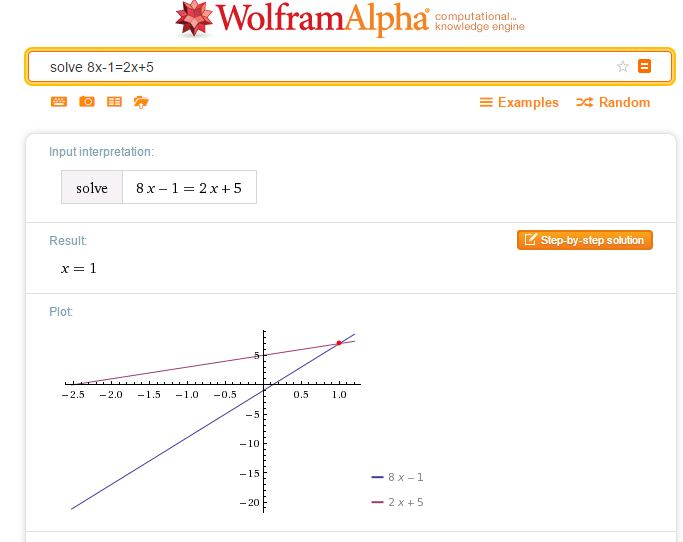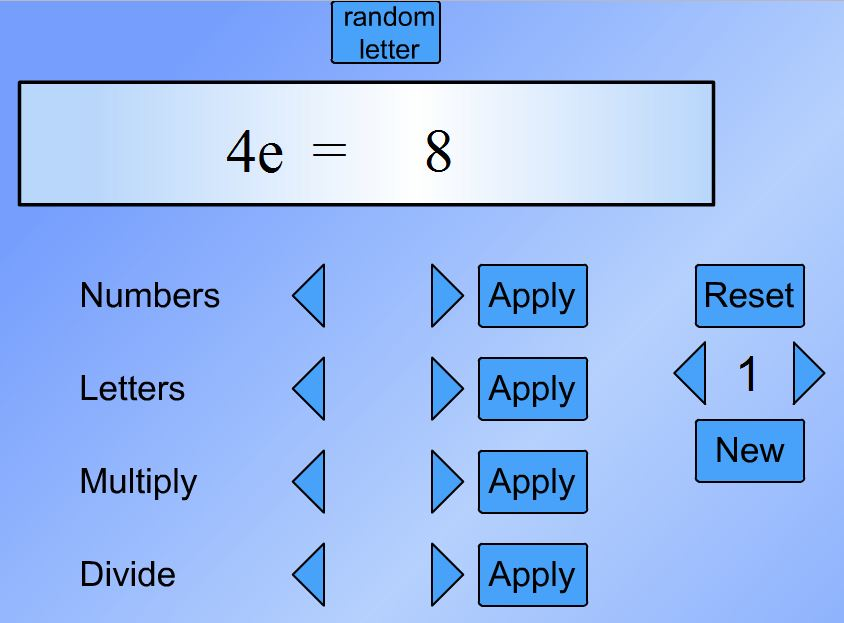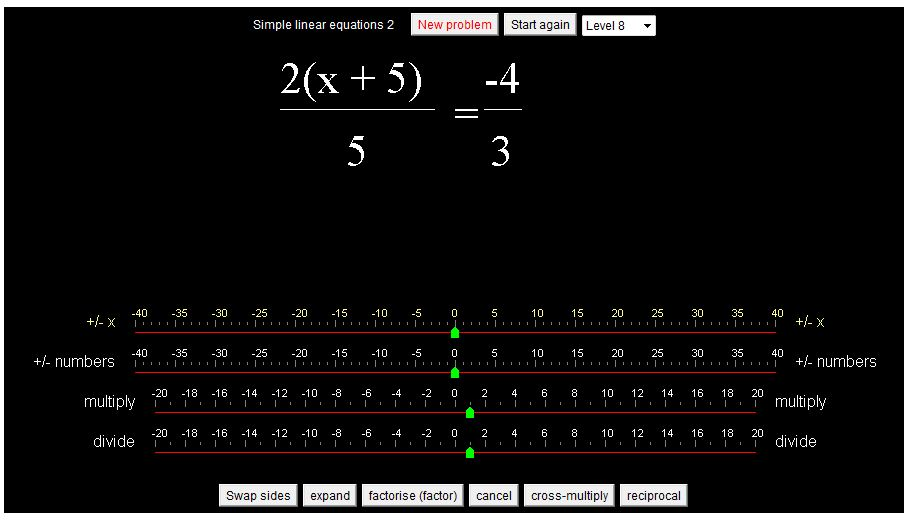# Solving Equations

A collection of resources to use to demonstrate and practise solving equations.A simple way to check a solution to an equation of any type is to simply enter your equation as a WolframAlpha query. Note that WolframAlpha includes a graphical illustration; it is so important for students to understand how equations may be solved graphically; I always illustrate graphical solutions when we are working with Algebra to help students make these links. Desmos of course, is ideal here.The following resources allow practice in choosing the correct operations to reach a solution.

Centre for Innovation in Mathematics Teaching
CIMT
have tutorials on equations: Linear Equations 1, Linear Equations 2, and Linear Equations with Brackets in their Interactive ResourcesChoose the type of equation you require then the sequence of operations required to solve the equation.
Select Do it after each operation, for example -32 Do it were the keys selected to start the above problem.

The next sites work in a similar way to the subtangent resource.

Flashy MathsSolving Equations (select the swf file to play online)There are 4 levels to choose from: the level 1 equations are of the type ax = b,
level 2, x±c = d, level 3, ex±f = g and level 4, hx±i = hx±j = k.
Choose the series of operations you require to solve the equation selecting apply at each stage to see the result of your chosen operation.
Choose numbers to add and subtract integers and letters to add and subtract variables.

MathsNetEquation Buster
Playing at level 5 introduces equations with fractions.From the National Library of Virtual Manipulatives try Algebra Balance Scales and Algebra Balance Scales – Negatives.
Note that these manipulatives allow you to create a problem of your own (so long as it has an integer solution) as well as solving problems given.From WaldoMaths, try this applet on solving simple linear equations and this on equations requiring more complex manipulation.
Each applet has several levels.Unlike the other resources this allows the user to enter the equation resulting from the chosen operation.
The solution is checked at each stage.
As well as solving the given problems (20 problems) the user can select ‘Make an equation yourself’ to enter any equation then solve that equation.
(Unlike the NLVM resource above the x can be any real number).

The following video shows the keystrokes required to enter and solve an equation.